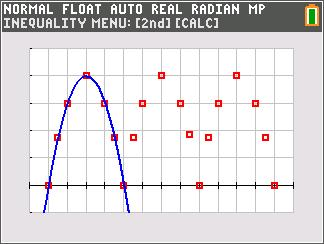### Bridge On The River Quad

Students graph a quadratic function that models the shape of a bridge trestle and then solve the related quadratic equation by completing the square.
•TI-84 Plus CE
•TI-84 Plus C Silver Edition
• TI-84 Plus Silver Edition
• TI-84 Plus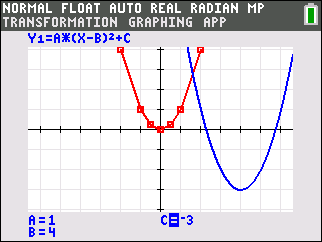Students graph quadratic functions and study how the constants in the equations compare to the coordinates of the vertices and the axes of symmetry in the graphs. The first part of the activity focuses on the vertex form, while the second part focuses on the standard form. Both activities include opportunities for students to pair up and play a graphing game to test how well they really understand the equations of quadratic functions.
•TI-84 Plus CE
•TI-84 Plus C Silver Edition
• TI-84 Plus Silver Edition
• TI-84 Plus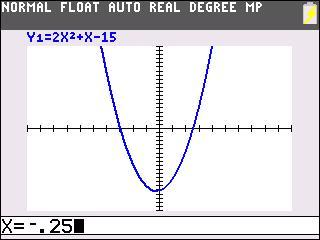### Using Symmetry to Find the Vertex of a Parabola

Students graph a quadratic function and investigate its symmetry by choosing pairs of points with the same y-value.
•TI-84 Plus CE
•TI-84 Plus C Silver Edition
• TI-84 Plus Silver Edition
• TI-84 Plus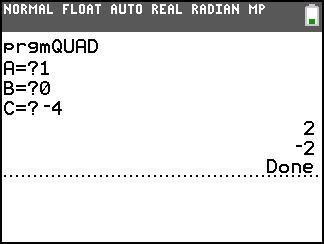Students make connections between the visual ways to find zeros of a parabola and algebraic ways with an emphasis on the quadratic formula.
•TI-84 Plus CE
•TI-84 Plus C Silver Edition
• TI-84 Plus Silver Edition
• TI-84 Plus### Applications of Parabolas

Students look for both number patterns and visual shapes that go along with quadratic relationships.
• TI-84 Plus Silver Edition
• TI-84 Plus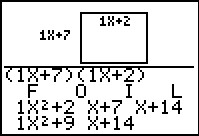### Area "FOILed" Again!

Students practice finding rectangular areas with algebraic expressions for the lengths of the sides.
• TI-84 Plus Silver Edition
• TI-84 Plus### Factoring Special Cases

Given a set of shapes whose combined areas represent the left-hand expression, students manipulate them to create rectangles whose areas are equal to the right-hand expression.
•TI-84 Plus CE
•TI-84 Plus C Silver Edition
• TI-84 Plus Silver Edition
• TI-84 Plus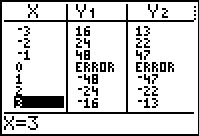### The Box Method

Students discover how to factor a quadratic function using the Box Method.
• TI-84 Plus Silver Edition
• TI-84 Plus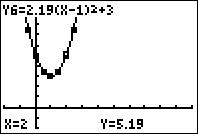### Manual Fit

Students manipulate parabolas so that the curve matches a set of data points.
•TI-84 Plus CE
•TI-84 Plus C Silver Edition
• TI-84 Plus Silver Edition
• TI-84 Plus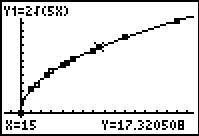### STOP

Students use an interactive page to calculate the speed of the car, given a stopping distance, and then approximate stopping distance, given the rate of the car.
•TI-84 Plus CE
•TI-84 Plus C Silver Edition
• TI-84 Plus Silver Edition
• TI-84 Plus••